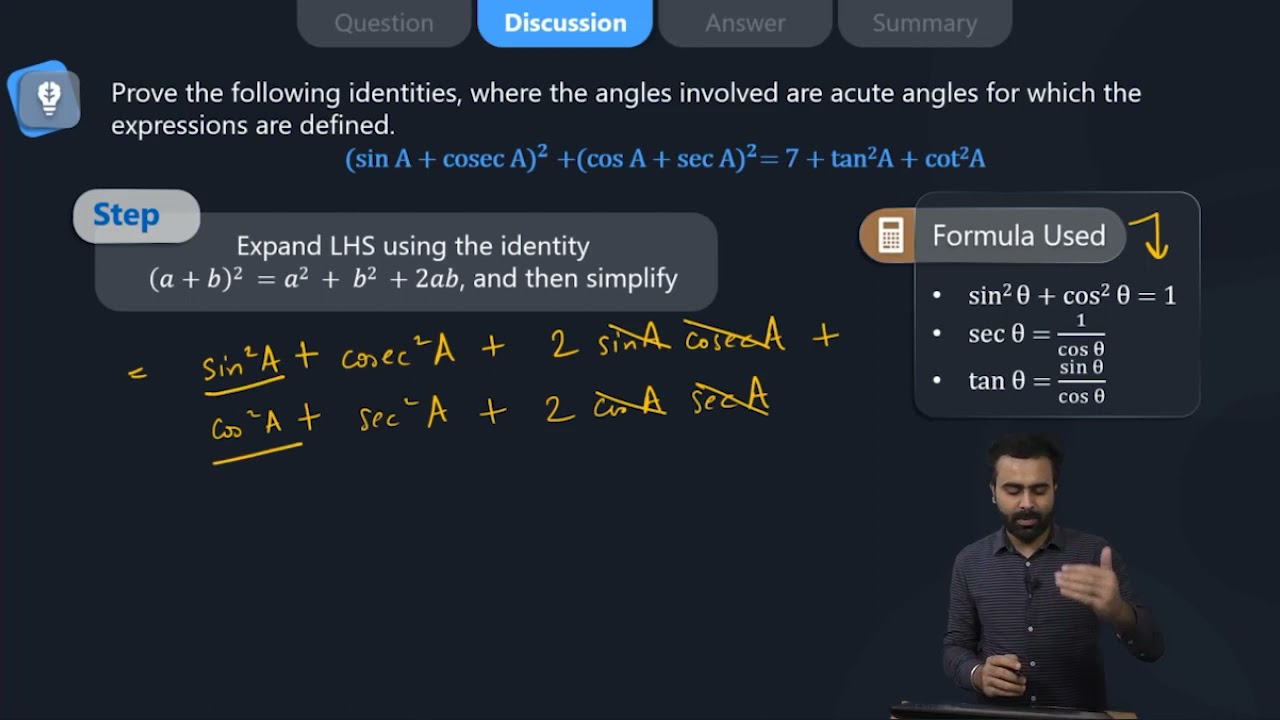Prove the following identity, where the angles involved are acute angles for which the expression is defined. (viii) (sin A + cosec A)^2 + (cos A + sec A)^2 = 7+tan^2 A+cot^2 A(sin A + cosec A)2 + (cos A + sec A)2 = 7 + tan2A + cot2A

L.H.S. = (sin A + cosec A)2 + (cos A + sec A)2

It is of the form (a+b)2, expand it

(a + b)2 = a2 + b2 +2ab

(sin A + cosec A)2 + (cos A + sec A)2 = (sin2A + cosec2A + 2 sin A cosec A) + (cos2A + sec2A + 2 cos A sec A)

(sin A + cosec A)2 + (cos A + sec A)2 = (sin2A + cos2A) + 2 sin A(1/sin A) + 2 cos A(1/cos A) + 1 + tan2A + 1 + cot2A

LHS = 1 + 2 + 2 + 2 + tan2A + cot2A

LHS = 7+tan2A+cot2A = R.H.S.

Therefore, (sin A + cosec A)2 + (cos A + sec A)2 = 7 + tan2A + cot2A

Hence proved.(3)(0)